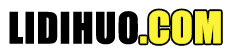# 归并排序

## 归并排序是如何工作的？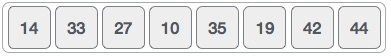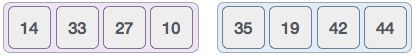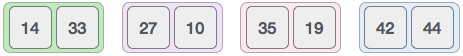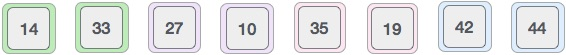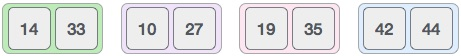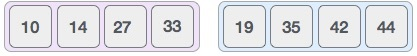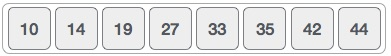### 算法

```Step 1 − if it is only one element in the list it is already sorted, return.
Step 2 − divide the list recursively into two halves until it can no more be divided.
Step 3 − merge the smaller lists into new list in sorted order.
```

### 伪代码

```procedure mergesort( var a as array )
if ( n == 1 ) return a
var l1 as array = a ... a[n/2]
var l2 as array = a[n/2+1] ... a[n]
l1 = mergesort( l1 )
l2 = mergesort( l2 )
return merge( l1, l2 )
end procedure
procedure merge( var a as array, var b as array )
var c as array
while ( a and b have elements )
if ( a > b )
add b to the end of c
remove b from b
else
add a to the end of c
remove a from a
end if
end while

while ( a has elements )
add a to the end of c
remove a from a
end while

while ( b has elements )
add b to the end of c
remove b from b
end while

return c

end procedure
```